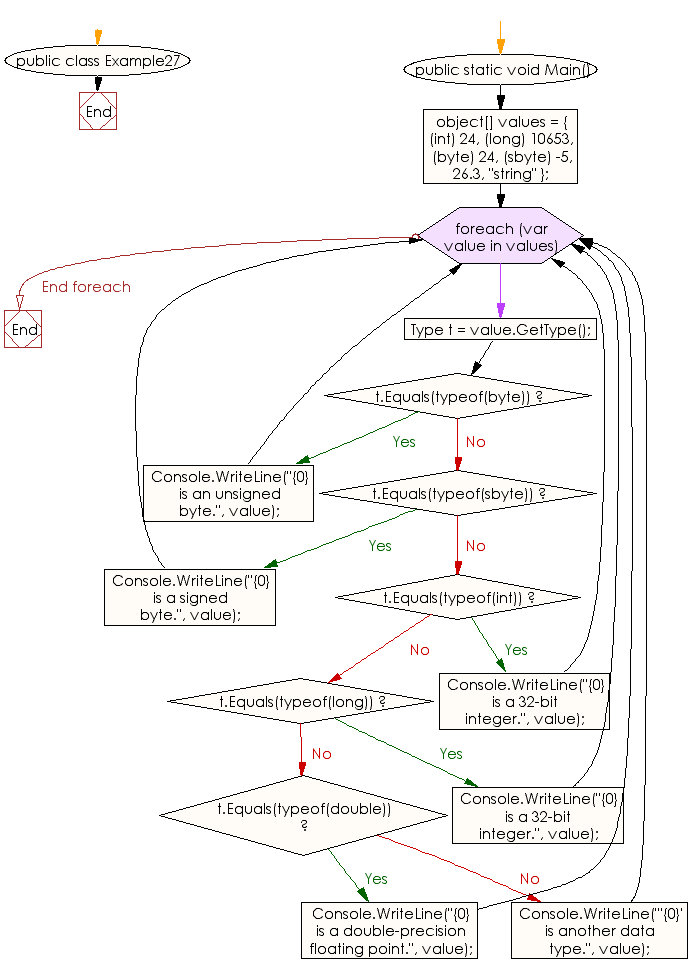﻿ C# - Determine the type of a particular object - w3resource# C# Sharp Exercises: Determine the type of a particular object

## C# Sharp DateTime: Exercise-27 with Solution

Write a C# Sharp program to determine the type of a particular object.

Sample Solution:-

C# Sharp Code:

``````using System;

public class Example27
{
public static void Main()
{
object[] values = { (int) 24, (long) 10653, (byte) 24, (sbyte) -5,
26.3, "string" };
foreach (var value in values) {
Type t = value.GetType();
if (t.Equals(typeof(byte)))
Console.WriteLine("{0} is an unsigned byte.", value);
else if (t.Equals(typeof(sbyte)))
Console.WriteLine("{0} is a signed byte.", value);
else if (t.Equals(typeof(int)))
Console.WriteLine("{0} is a 32-bit integer.", value);
else if (t.Equals(typeof(long)))
Console.WriteLine("{0} is a 32-bit integer.", value);
else if (t.Equals(typeof(double)))
Console.WriteLine("{0} is a double-precision floating point.",
value);
else
Console.WriteLine("'{0}' is another data type.", value);
}
}
}
```
```

Sample Output:

```24 is a 32-bit integer.
10653 is a 32-bit integer.
24 is an unsigned byte.
-5 is a signed byte.
26.3 is a double-precision floating point.
'string' is another data type.
```

Flowchart :C# Sharp Code Editor:

Improve this sample solution and post your code through Disqus

What is the difficulty level of this exercise?

Test your Programming skills with w3resource's quiz.

﻿